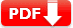# OGET Math Practice Test 2020 Question Answers [PDF]

OGET Oklahoma General Education Test (074) Mathematics Practice Test Question Answers 2020. You can download OGET Math Practice Test printable and editable PDF for exam preparation.

 Practice Test Name OGET Test Prep Test Type Math Practice Test 1 Question Type Multiple Choice Topics Covered Algebra and Geometry Difficulty Level High School Printable/Editable File Available Yes PDF & DOC Total Question 10 Prerequisite NONE

## OGET Math Practice Test-1

 Q1. Simplify the expression by combine similar terms 8 – 5(6x – 2) 🔘 A.  3 – 12x 🔘 B.  3 – 28x 🔘 C.  18 – 30x 🔘 D. 3 – 4x
Answer: C.  18 – 30x (Sol: 8 – 30x + 10 18 – 30x)
 Q2. Which of the following expressions is equivalent to the expression 17 − 4x for all values of x? 🔘 A. 1 – (2x – 8) 🔘 B. 3 + (3x – 6) 🔘 C. 5 – (4x – 12) 🔘 D. 5 + (-6x – 17)
Answer:C 5 – (4x – 12) (Sol: 5 – 4x + 12 17 – 4x )
 Q3. If \frac34 x – 11 = 1 then find the value of x 🔘 A. 2 🔘 B. 4 🔘 C. 8 🔘 D. 16
 Q4.  Find the side z is between which two integers in the following figure?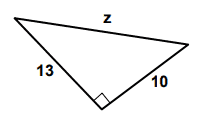🔘 A. 15 and 16 🔘 B. 16 and 17 🔘 C. 18 and 19 🔘 D. 20 and 21
Answer:B 16 and 17 (hints: use The Pythagorean Theorem a2+b2=c2 132+102 = c2
 Q5. Find the vaule of a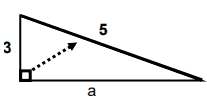🔘 A.  1 🔘 B. 2 🔘 C. 3 🔘 D. 4
Answer:D 4  (use Pythagorean Theorem a2+b2=c2 )
 Q6. James has twice as many coins as Robert. Joseph has 5 more coins than James. If the total number of coins is 50, how many coins does Joseph have? 🔘 A. 23 🔘 B. 26 🔘 C. 30 🔘 D. 34

Let C = # of coins James has
Let 2C =
# of coins Robert has
Let 2C + 5 =
# of coins Joseph has

C + 2C + 2C + 5 = 50
5C + 5 = 50
5C = 45
C = 9

James has 9 coins
Robert has 2(9) = 18 coins
Joseph has 18 + 5 = 23 coins

 Q7. The table shows how many students entered class at different times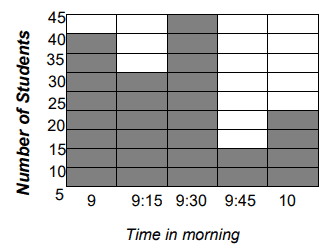How many students entered class after 9 a.m.? 🔘 A. 80 🔘 B. 90 🔘 C. 95 🔘 D. 105

Notice it says AFTER 9 a.m.
30 at 9:15 a.m.
45 at 9:30 a.m.
10 at 9:45 a.m.
20 at 10:00 a.m.
Total = 30 + 45 + 10 + 20 = 105

 Q8. The following graph of line k is shown in the xy-plane above. The point on line k that has x-coordinate 40 is not shown. What is the y-coordinate of that point?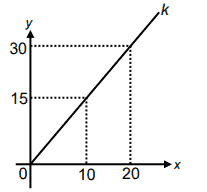🔘 A. 35 🔘 B. 40 🔘 C. 55 🔘 D. 60

X increases by 10 so you would have to move 2 more on the x axis.
This means you would have to move 2 up on the y-axis. Y increases by 15 so => 30 + 15 + 15 = 60

 Q9. What is the length of a diagonal in the following rectangle?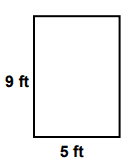🔘 A. 8.5 ft 🔘 B. 9.6 ft 🔘 C. 10.3 ft 🔘 D. 12.9 ft
 Q10. Michele sells advertising in a local magazine. She makes $450 per week plus$50 for every new full page ad she sells that week. If she wants to make at least \$3000 so she can take a trip to Bali, how many ads will she need to sell? 🔘 A. 25 🔘 B. 31 🔘 C. 50 🔘 D. 51
 Document Type Download Link Free Editable Doc File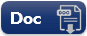Free Printable PDF File